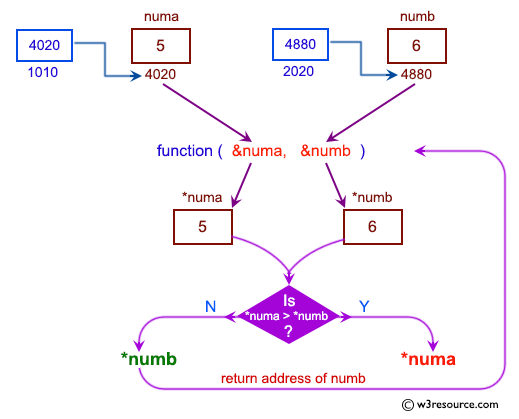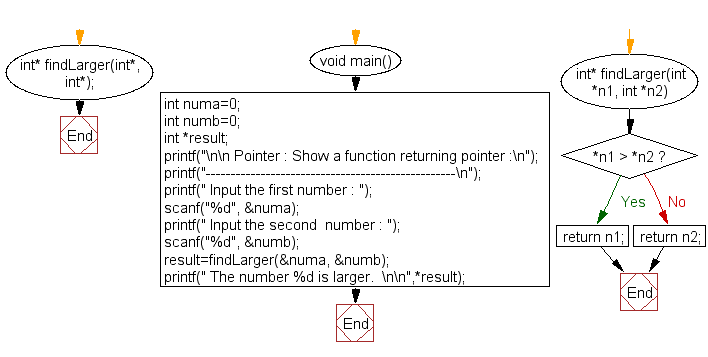﻿ C exercises: Show a function returning pointer - w3resource# C Exercises: Show a function returning pointer

## C Pointer : Exercise-15 with Solution

Write a program in C to show how a function returning pointer.

Pictorial Presentation:Sample Solution:

C Code:

``````#include <stdio.h>
int* findLarger(int*, int*);
void main()
{
int numa=0;
int numb=0;
int *result;
printf("\n\n Pointer : Show a function returning pointer :\n");
printf("--------------------------------------------------\n");
printf(" Input the first number : ");
scanf("%d", &numa);
printf(" Input the second  number : ");
scanf("%d", &numb);

result=findLarger(&numa, &numb);
printf(" The number %d is larger.  \n\n",*result);
}

int* findLarger(int *n1, int *n2)
{
if(*n1 > *n2)
return n1;
else
return n2;
}
```
```

Sample Output:

``` Pointer : Show a function returning pointer :
--------------------------------------------------
Input the first number : 5
Input the second  number : 6
The number 6 is larger.
```

Flowchart:C Programming Code Editor:

Have another way to solve this solution? Contribute your code (and comments) through Disqus.

What is the difficulty level of this exercise?

﻿

New Content: Composer: Dependency manager for PHP, R Programming